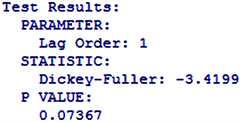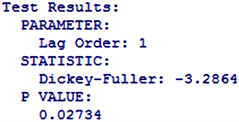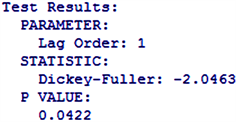CPI指数，ARIMA(pdq)模型，预测, CPI Index ARIMA(pdq) Model Forecast1. 研究背景、意义及目的

2. 模型介绍

{ ϕ ( B ) Δ d x t = θ ( B ) ϵ t E ( ϵ t ) = 0 ,   V a r ( ϵ t ) = σ ϵ 2 ,   E ( ε t ϵ s ) = 0 ,   s ≠ t E ( ε S ϵ t ) = 0 ,   ∀ s < t

Δ d x t = ∑ i = 0 d ( − 1 ) i C d i x t − i

Δ x t = γ 1 x t − 1 + … + γ p x t − p + ϵ t ,     t = 1 , 2 , ⋯ , T (1)

Δ x t = μ + γ 1 x t − 1 + ⋯ + γ p x t − p + ϵ t ,     t = 1 , 2 , ⋯ , T (2)

Δ x t = μ + β t + γ 1 x t − 1 + ⋯ + γ p x t − p + ϵ t ,     t = 1 , 2 , ⋯ , T (3)

H 0 : γ 1 = 0

H 1 : γ 1 ≠ 0

3. 数据来源

4. 实证分析4.1. 数据处理

4.2. 随机性检验

4.3. 平稳性检验4.4. 模型拟合

x t = x t − 1 + ε t + 0.8186 ε t − 1

4.5. 模型的显著性检验

4.6. 预测

5. 结论